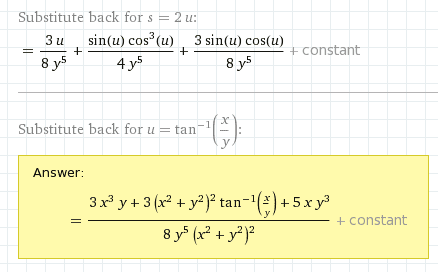# Trig Substitution

thercias

## Homework Statement## The Attempt at a Solution

This isn't really a traditional question, but can someone explain to me how substituting u = tan^-1(x/y) got to that final value? I'm trying to understand this for an exam coming up.

## Answers and Replies

Homework Helper
I didn't check, but using these identities
$$\sin(\tan^{-1}(z)) = \frac{z}{\sqrt{z^2 + 1}}$$
and
$$\cos(\tan^{-1}(z)) = \frac{1}{\sqrt{z^2 + 1}}$$
should get you started.

Homework Helper
Gold Member
Dearly Missed
It is a good exercise to derive the simplified expressions of trigs with inverse trigs as their arguments.
For example, let's take cos(asin(x)). Not bothering about the precise domain for the inverse trig right now, we know that we have the identity:
$$\cos^{2}(asin(x))+\sin^{2}(asin(x))=1$$
But, the latter term on the LHS simplifes to x^2!!
Thus, we have:
$$\cos(asin(x))=\pm\sqrt{1-x^{2}}$$

This is also readily seen geometrically:
If we look at a right-angled triangle with unit hypotenuse, and sine equal to x (to which the relevant angle is asin(x)), then that expression falls right out of the Pythagorean theorem.